Function Repository Resource:

# OrderedTupleIndex

Give the index of an ordered tuple

Contributed by: Ed Pegg Jr
 ResourceFunction["OrderedTupleIndex"][tuple] gives the index of the ordered tuple.

## Details and Options

Ordered tuples are considered to be lists {a1,a2,,ak} such that the ai are non-negative and non-decreasing.
For any ordered tuple, a unique index n can be defined via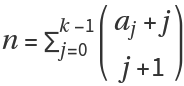.
For example, subset {1, 2, 2} has index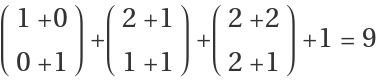.

## Examples

### Basic Examples (2)

The following ordered 3-tuple sequence can be extended to infinity:

 In:=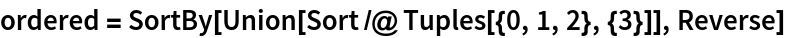Out=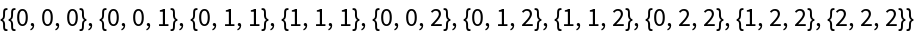The function returns indices of one to ten for these ordered tuples:

 In:=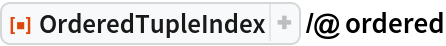Out=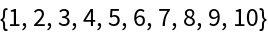Any nondecreasing list of nonnegative integers can be considered as an ordered tuple with a unique index:

 In:=Out=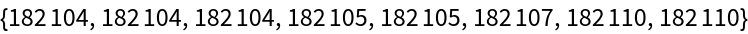The result above is an ordered tuple with a unique index:

 In:=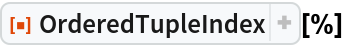Out=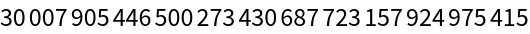### Scope (3)

Here are some ordered 2-tuples with their indices to show their structure:

 In:=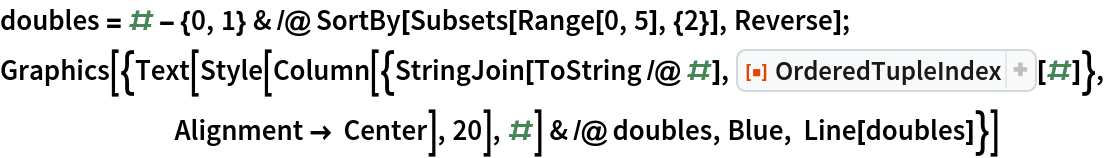Out=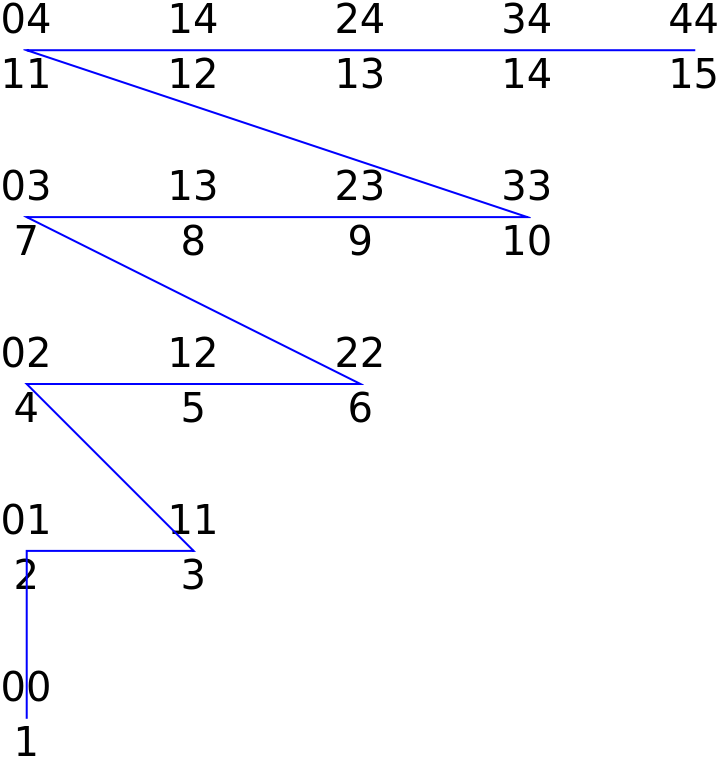The ordered 2-tuple {4,4} has an index of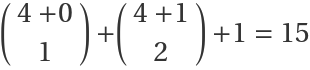:

 In:=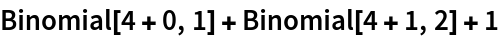Out=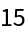OrderedTupleIndex returns the same thing:

 In:=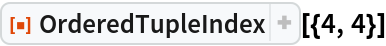Out=The structure of ordered 3-tuples in 3D:

 In:=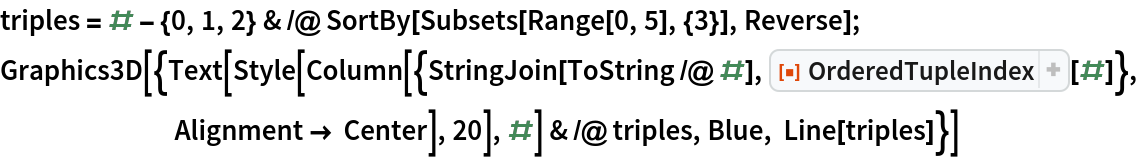Out=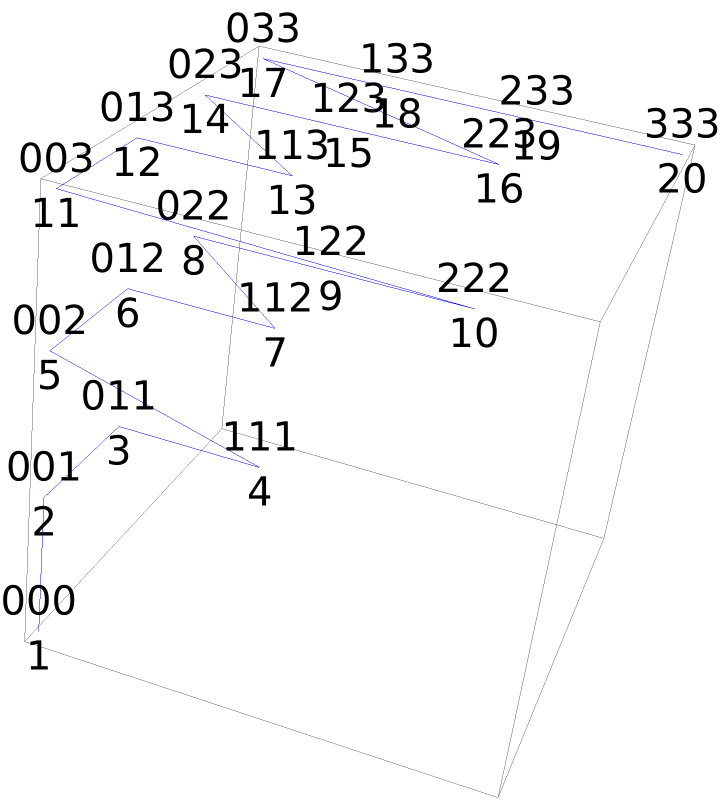### Properties and Relations (3)

Use Tuples to produce ordered 3-tuples:

 In:=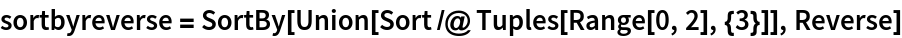Out=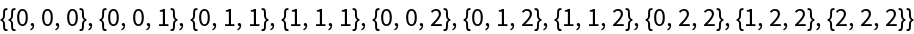The same sequence of ordered 3-tuples can be obtained from ordered Subsets:

 In:=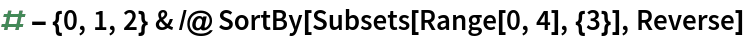Out=Indices of this sequence:

 In:=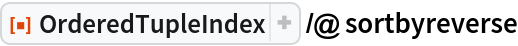Out=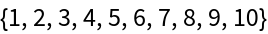## Version History

• 1.0.0 – 06 December 2019